# Methods and formulas for goodness-of-fit statistics in Analyze Variability

## S

### Notation

TermDescription
MSEmean square error

## R-sq

R2 is also known as the coefficient of determination.

### Notation

TermDescription
yi i th observed response valuemean responsei th fitted response

While the calculations for adjusted R2 can produce negative values, Minitab displays zero for these cases.

### Notation

TermDescription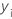ith observed response value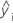ith fitted responsemean response
nnumber of observations
pnumber of terms in the model

## R-sq (pred)

While the calculations for R2(pred) can produce negative values, Minitab displays zero for these cases.

### Notation

TermDescription
yi i th observed response value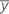mean response
n number of observations
ei i th residual
hi i th diagonal element of X(X'X)–1X'
X design matrix

## PRESS

### Notation

TermDescription
n number of observations
ei i th residual
hi i th diagonal element of X(X'X)–1X'

## Log-likelihood

The calculation of the log-likelihood depends on the estimation method for the analysis.

### Least squares estimation

Minitab uses the following equation for the log-likelihood:
The weights for the analysis are either the specified weights or given by the following equation:

### Maximum likelihood estimation

This calculation of the log-likelihood assumes that the standard deviations are from normal distributions and that the natural log of the standard deviation follows a linear model.

### Notation

TermDescription
nthe number of rows with no missing data
Rthe sum of squares for error for the model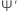the trigamma function
vithe degrees of freedom for the ith standard deviationthe gamma function
Sithe ith sample standard deviation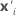the row of the design matrix associated with the ith standard deviation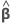the maximum likelihood estimates of the model coefficients

## AICc (Akaike's Corrected Information Criterion)

AICc is not calculated when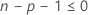.

### Notation

TermDescription
pthe number of coefficients in the model, including the constant
nthe number of rows in the data with no missing data

## BIC (Bayesian Information Criterion)

### Notation

TermDescription
pthe number of coefficients in the model, not counting the constant
nthe number of rows in the data with no missing data

## Mallows' Cp

### Notation

TermDescription
SSEpsum of squared errors for the model under consideration
MSEmmean square error for the model with all candidate terms
nnumber of observations
pnumber of terms in the model, including the constant
By using this site you agree to the use of cookies for analytics and personalized content.  Read our policy